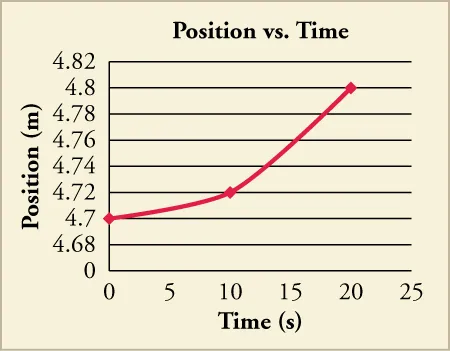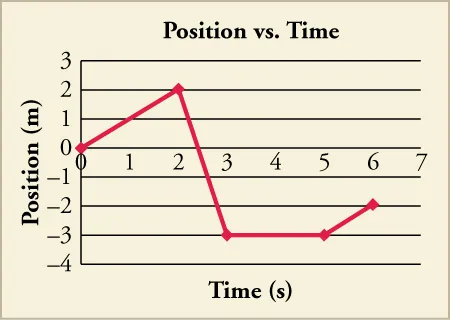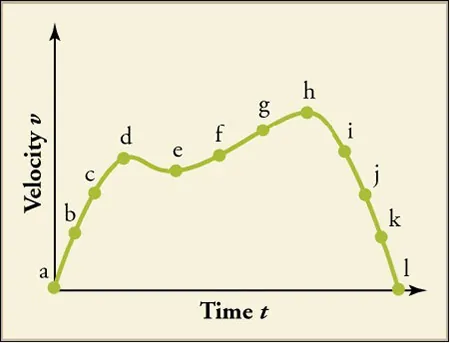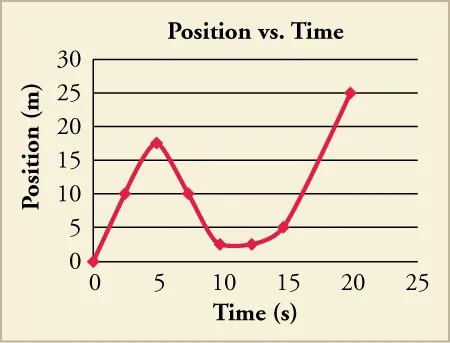Physics

# Extended Response

PhysicsExtended Response

### Extended Response

#### 2.1Relative Motion, Distance, and Displacement

53.

Find the distance traveled from the starting point for each path.

Which path has the maximum distance?

1. The distance for Path A is 6 m, Path B is 4 m, Path C is 12 m and for Path D is 7 m. The net displacement for Path A is 7 m, Path B is –4m, Path C is 8 m and for Path D is –5m. Path C has maximum distance and it is equal to 12 meters.
2. The distance for Path A is 6 m, Path B is 4 m, Path C is 8 m and for Path D is 7 m. The net displacement for Path A is 6 m, Path B is –4m, Path C is 12 m and for Path D is –5 m. Path A has maximum distance and it is equal to 6 meters.
3. The distance for Path A is 6 m, Path B is 4 m, Path C is 12 m and for Path D is 7 m. The net displacement for Path A is 6 m, Path B is –4 m, Path C is 8 m and for Path D is –5 m. Path C has maximum distance and it is equal to 12 meters.
4. The distance for Path A is 6 m, Path B is –4 m, Path C is 12 m and for Path D is –5 m. The net displacement for Path A is 7 m, Path B is 4 m, Path C is 8 m and for Path D is 7 m. Path A has maximum distance and it is equal to 6 m.
54 .
Alan starts from his home and walks 1.3 km east to the library. He walks an additional 0.68 km east to a music store. From there, he walks 1.1 km north to a friend’s house and an additional 0.42 km north to a grocery store before he finally returns home along the same path. What is his final displacement and total distance traveled?
1. Displacement is 0 km and distance is 7 km.
2. Displacement is 0 km and distance is 3.5 km.
3. Displacement is 7 km towards west and distance is 7 km.
4. Displacement is 3.5 km towards east and distance is 3.5 km.

#### 2.2Speed and Velocity

55 .
Two runners start at the same point and jog at a constant speed along a straight path. Runner A starts at time t = 0 s, and Runner B starts at time t = 2.5 s. The runners both reach a distance 64.0 m from the starting point at time t = 25 s. If the runners continue at the same speeds, how far from the starting point will each be at time t = 45 s?
1. Runner A will be $72 \times 10^3$ m away and Runner B will be $59.5 \times 10^3$ m away from the starting point.
2. Runner A will be $1.2 \times 10^2$ m away and runner B will be $1.1 \times 10^2$ m away from the starting point.
3. Runner A will be $1.15 \times 10^2\,\text{m}$ away and Runner B will be $1.21 \times 10^2\,\text{m}$ away from the starting point.
4. Runner A will be $7.2 \times 10^2\,\text{m}$ away and Runner B will be $1.3 \times 10^2\,\text{m}$ away from the starting point.
56.

A father and his daughter go to the bus stop that is located 75 m from their front door. The father walks in a straight line while his daughter runs along a varied path. Despite the different paths, they both end up at the bus stop at the same time. The father’s average speed is 2.2 m/s, and his daughter’s average speed is 3.5 m/s. (a) How long does it take the father and daughter to reach the bus stop? (b) What was the daughter’s total distance traveled? (c) If the daughter maintained her same average speed and traveled in a straight line like her father, how far beyond the bus stop would she have traveled?

1. (a) 21.43 s (b) 75 m (c) 0 m
2. (a) 21.43 s (b) 119 m (c) 44 m
3. (a) 34 s (b) 75 m (c) 0 m
4. (a) 34 s (b) 119 m (c) 44 m

#### 2.3Position vs. Time Graphs

57.

What kind of motion would create a position graph like the one shown?1. uniform motion
2. any motion that accelerates
3. motion that stops and then starts
4. motion that has constant velocity
58.

What is the average velocity for the whole time period shown in the graph?1. $– 1 3 m/s – 1 3 m/s$
2. $– 3 4 m/s – 3 4 m/s$
3. $1 3 m/s 1 3 m/s$
4. $3 4 m/s 3 4 m/s$

#### 2.4Velocity vs. Time Graphs

59.

Consider the motion of the object whose velocity is charted in the graph.During which points is the object slowing down and speeding up?

1. It is slowing down between d and e. It is speeding up between a and d and e and h
2. It is slowing down between a and d and e and h. It is speeding up between d and e and then after i.
3. It is slowing down between d and e and then after h. It is speeding up between a and d and e and h.
4. It is slowing down between a and d and e and h. It is speeding up between d and e and then after i.
60.

Divide the graph into approximate sections, and use those sections to graph the velocity vs. time of the object.Then calculate the acceleration during each section, and calculate the approximate average velocity.

1. Acceleration is zero and average velocity is 1.25 m/s.
2. Acceleration is constant with some positive value and average velocity is 1.25 m/s.
3. Acceleration is zero and average velocity is 0.25 m/s.
4. Acceleration is constant with some positive value and average velocity is 0.25 m/s.
Order a print copy

As an Amazon Associate we earn from qualifying purchases.